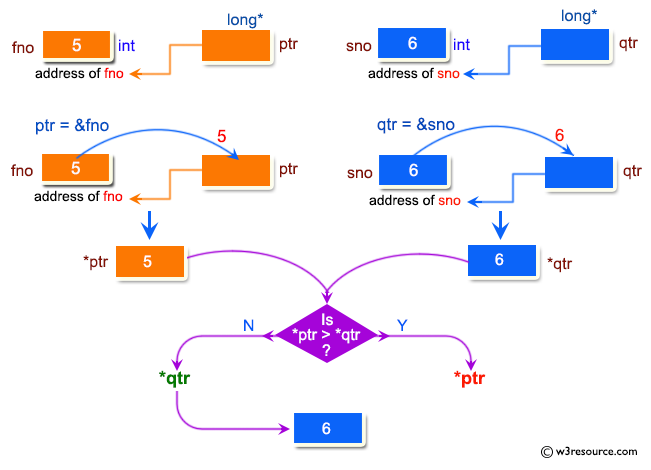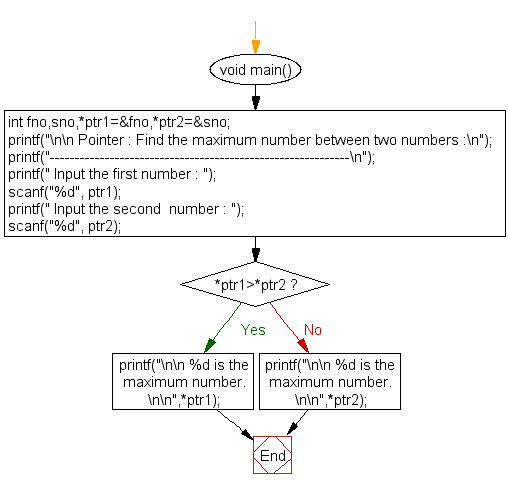﻿ C Program: Find the maximum number between two numbers - w3resource# C Exercises: Find the maximum number between two numbers

## C Pointer : Exercise-6 with Solution

Write a program in C to find the maximum number between two numbers using a pointer.

Pictorial Presentation:Sample Solution:

C Code:

``````#include <stdio.h>
#include <stdlib.h>
void main()
{
int fno,sno,*ptr1=&fno,*ptr2=&sno;

printf("\n\n Pointer : Find the maximum number between two numbers :\n");
printf("------------------------------------------------------------\n");

printf(" Input the first number : ");
scanf("%d", ptr1);
printf(" Input the second  number : ");
scanf("%d", ptr2);

if(*ptr1>*ptr2)
{
printf("\n\n %d is the maximum number.\n\n",*ptr1);
}
else
{
printf("\n\n %d is the maximum number.\n\n",*ptr2);
}

}
```
```

Sample Output:

``` Pointer : Find the maximum number between two numbers :
------------------------------------------------------------
Input the first number : 5
Input the second  number : 6

6 is the maximum number.
```

Flowchart:C Programming Code Editor:

Have another way to solve this solution? Contribute your code (and comments) through Disqus.

What is the difficulty level of this exercise?

Test your Programming skills with w3resource's quiz.

﻿

## C Programming: Tips of the Day

How to format strings using printf() to get equal length in the output?

You can specify a width on string fields, e.g.

`printf("%-20s", "initialization..."); `

And then whatever's printed with that field will be blank-padded to the width you indicate.

The - left-justifies your text in that field.

Ref : https://bit.ly/34DMOc3